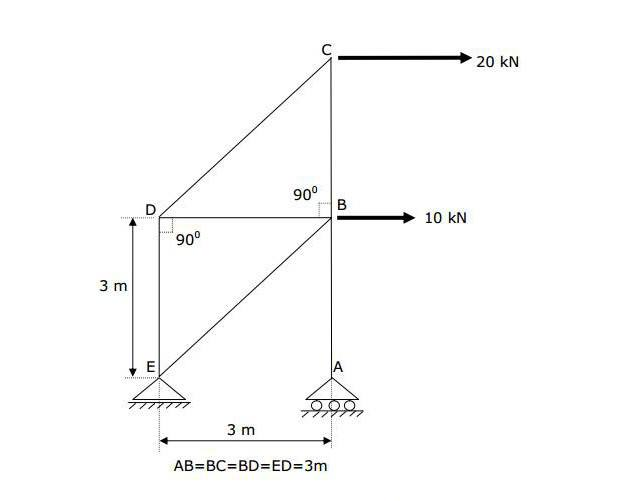# Moments Forces Help

How do I determine the support reactions at A & E in this example?Help is much appreciated.

SteamKing
Staff Emeritus
Homework Helper
Have you tried writing equations of static equilibrium for this frame?

Have you tried writing equations of static equilibrium for this frame?

How?

SteamKing
Staff Emeritus
Homework Helper
Have you written equations of static equilibrium for any structure before?

Have you written equations of static equilibrium for any structure before?

Right I've worked it out.

I've got E vertical as 50kN.

A horizontal as -30kN, vertical as -50kN.

Only problem is the answers have them listed as these values but I have all of the signs wrong.

SteamKing
Staff Emeritus
Homework Helper
Right I've worked it out.

I've got E vertical as 50kN.

A horizontal as -30kN, vertical as -50kN.

Only problem is the answers have them listed as these values but I have all of the signs wrong.
This indicates that the direction in which you assumed the reactions are acting is opposite of the direction in which they act.

This indicates that the direction in which you assumed the reactions are acting is opposite of the direction in which they act.

Yeah, I get that, I'm unsure why the answer is in correct.

Here's my working for vertical E:

∑M = (10 x 3) + (20 x 6) - (E x 3)
= 150 - 3E
Ev = 50

The correct answer is - 50

Any idea where I've gone wrong?

Thanks.

SteamKing
Staff Emeritus
Homework Helper
If you don't set up a convention for what constitutes a positive direction for forces and a positive orientation for moments, your equilibrium equations often will give you incorrect signs in the results.

It helps to write both equilibrium equations. There should be an equation for the forces such that ∑F = 0, just as there is one for the moments, ∑M = 0.

CWatters
Homework Helper
Gold Member
∑M = (10 x 3) + (20 x 6) - (E x 3)

What SteamKing said. Its much better to write...

I define clockwise as +ve and then

(+10 x 3) + (+20 x 6) + (?E x 3) = 0

Note: I have explicitly written the sign in front of the 10 and 20N. The "+" sign before the E is because I am summing the moments, it is NOT because I have assumed E has any particular direction. To further clarify I have put a ? indicating the sign of E is unknown. I wouldn't normally put a ? mark but you can if it helps.

Rearrange

?E = - {(+10 x 3) + (+20 x 6)} / 3

?E = - 50

So the unknown sign of E is -ve. That means E acts anticlockwise (eg downwards).

Last edited: